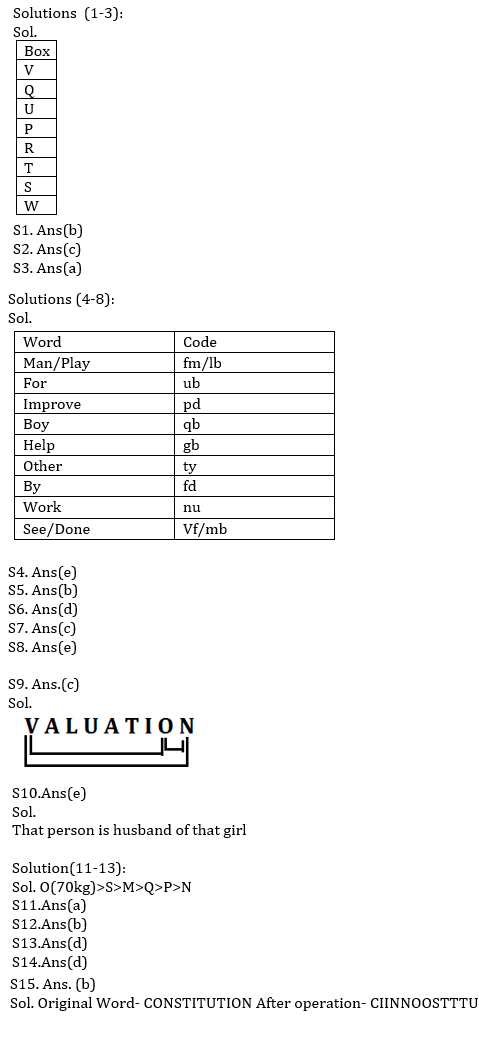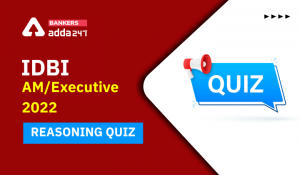Latest Banking jobs   »   Reasoning Ability Quiz For IBPS Clerk/NIACL...

# Reasoning Ability Quiz For IBPS Clerk/NIACL AO Prelims 2021- 28th August

Directions (1-5): Study the following information carefully and answer the given questions.
Eight boxes P, Q, R, S, T, U, V and W are placed one above the another, but not necessarily in the same order. Two boxes are placed between U and T. U is placed above T. One box is placed between U and V. Three boxes are placed between P and W. P is placed immediately below U. Two boxes are placed between R and W. Q is placed above S.

Q1. Which of the following Box is placed at top?
(a) Q
(b) V
(c) U
(d) W
(e) None of these

Q2. How many boxes are placed between Box T and Box W?
(a) None
(b) Two
(c) One
(d) Four
(e) Three

Q3. Which of the following Box is placed at bottom?
(a) W
(b) R
(c) T
(d) S
(e) None of these

Directions (4-8): Study the following information and answer the following questions:
In a certain code language-
‘man play for improve’ is written as ‘fm lb pd ub’,
‘boy for help other’ is written as ‘ub ty qb gb’,
‘improve other by work’ is written as ‘fd pd nu ty ’,
‘boy see work done’ is written as ‘qb nu vf mb’,

Q4. What is the code for ‘see’ in the given code language?
(a) qb
(b) vf
(c) nu
(d) mb
(e) Cannot determined

Q5. Which among the following word is written as ‘gb’ in the given code language?
(a) work
(b) help
(c) man
(d) boy
(e) None of these

Q6. Which among the following word is written as ‘fd pd’ in the given code language?
(a) play improve
(b) man done
(c) by work
(d) improve by
(e) None of these

Q7.What is the possible code for ‘other time’ in the given code language?
(a) wc fd
(b) mb vf
(c) ty wc
(d) rb mb
(e) ty ub

Q8. Which among the following word is written as ‘fm’ in the given code language?
(a) man
(b) boy
(c) done
(d) play
(e) Either (a) or (d)

Q9. How many pairs of letters are there in the word “VALUATION” each of which have as many letters between them (both forward and backward directions) in the word as they have between them in the English alphabetical series?
(a) None
(b) Two
(c) Three
(d) More than three
(e) None of these

Q10. Pointing towards photograph a person said “This girl is daughter in law of my father”. That person has no brother. How is person related to that girl?
(a) Father
(b) Father in law
(c) Grandfather
(d) Son
(e) None of these

Directions (11-13): Study the information carefully and answer the questions given below.
Six persons M, N, O, P, Q, and S are different weight. P is heavier than N. M is lighter than S. Q is heavier than N. M is not lighter than P and Q. S is not heaviest, and Q is not second lightest. The weight of heaviest is 70kg.

Q11. Who among the following person is third heaviest?
(a) M
(b) S
(c) P
(d) Q
(e) Can’t be determined

Q12. Who among the following is the lightest person?
(a) M
(b) N
(c) Q
(d) P
(e) None of these

Q13. If weight of M is 67kg what may be the weight of S?
(a) 60kg
(b) 63kg
(c) 61kg
(d) 69kg
(e) None of these

Q14. If in the number 39475132, 2 is multiply to each of the digit which is less than 4 and 3 is subtracted from each of the digit which is more than 4 and equal to 4 then how many digits are repeating in the number thus formed?
(a) None
(b) Four
(c) One
(d) Three
(e) Two

Q15. If all the letters in the word CONSTITUTION are arranged in alphabetical order from left to right, then how many alphabets remains same in their position?
(a) Three
(b) One
(c) None
(d) Two
(e) four

Solutions#### Congratulations!Incorrect details? Fill the form again here

•Reasoning Ability Quiz For IBPS RRB PO C...
•Reasoning Ability Quiz For IBPS Clerk Pr...
•Reasoning Ability Quiz For IBPS RRB PO P...
•Reasoning Ability Quiz For IDBI AM/Execu...
•Reasoning Ability Quiz For IBPS RRB PO P...
•Reasoning Ability Quiz For IDBI AM/Execu...